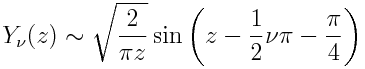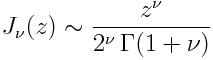# How to visualize Bessel functions

Bessel functions are sometimes called cylindrical functions because they arise naturally from physical problems stated in cylindrical coordinates. Bessel functions have a long list of special properties that make them convenient to use. But because so much is known about them, introductions to Bessel functions can be intimidating. Where do you start? My suggestion is to start with a few properties that give you an idea what their graphs look like.

A textbook introduction would define the Bessel functions and carefully develop their properties, eventually getting to the asymptotic properties that are most helpful in visualizing the functions. Maybe it would be better to learn how to visualize them first, then go back and define the functions and show they look as promised. This way you can have a picture in your head to hold onto as you go over definitions and theorems.

I’ll list a few results that describe how Bessel functions behave for large and small arguments. By putting these two extremes together, we can have a fairly good idea what the graphs of the functions look like.

There are two kinds of Bessel functions, J(z) and Y(z). Actually, there are more, but we’ll just look at these two. These functions have a parameter ν (Greek nu) written as a subscript. The functions Jν(z) are called “Bessel functions of the first kind” and the functions Yν are called … wait for it … “Bessel functions of the second kind.” (Classical mathematics is like classical music as far as personal names followed by ordinal numbers: Beethoven’s fifth symphony, Bessel functions of the first kind, etc.)

Roughly speaking, you can think of J‘s and Y‘s like cosines and sines. In fact, for large values of z, J(z) is very nearly a cosine and Y(z) is very nearly a sine. However, both are shifted by a phase φ that depends on ν and are dampened by 1 /√z . That is,  for large values of z, J(z) is roughly proportional to cos(z – φ)/√z and Y(z) is roughly proportional to sin(z – φ)/√z.

More precisely, as z goes to infinity, we haveandThese statements hold as long as |arg(z)| < π. The error in each is on the order O(1/|z|).

Now lets look at how the functions behave as z goes to zero. For small z, Jν behaves like zν and Yν behaves like z. Specifically,and for ν > 0,If ν = 0, we haveNow let’s use the facts above to visualize a couple plots.

First we plot J1 and J5. For large values of z, we expect J1(z) to behave like cos(z – φ) / √z where φ = 3π/4 and we expect J5(z) to behave like cos(z – φ) / √z where φ = 11π/4. The two phases differ by 2π and so the two functions should be nearly in phase.

For small z, we expect J1(z) to be roughly proportional z  and so its graph comes into the origin at an angle. We expect J5(z) to be roughly proportional to z5 and so its graph should be flat near the origin.

The graph below shows that the function graphs look like we might have imagined from the reasoning above. Notice that the amplitude of the oscillations is decreasing like 1/√z.

Next we plot J1 and Y1. For large arguments we would expect these functions to be a quarter period out of phase, just like cosine and sine, since asymptotically J1 is proportional to cos(z – 3π/4) / √z and Y1 is proportional to sin(z – 3π/4) / √z. For small arguments, J1(z) is roughly proportional to z and Y1(z) is roughly proportional to -1/z. The graph below looks as we might expect.

## 13 thoughts on “How to visualize Bessel functions”

1. Dagiwal

Hi John,
Hope you are fine. I am a Statistics student working on Gravitational waves’ detection problem, however my physics knowledge is same as an under graduate student, in fact I don’t have to work with pure gravity related theory. I want to ask the following.
How would you explain Bessel functions to a non Physicist/Mathematician
, as to why they are used (in the contexts of generating waves)?
I could not find any suitable explanation of it besides one that they
are used for solution of differential equations, but this is again a mathematical answer. I need a real world explanation, as to why we use Bessel functions?

2. Dagiwal, I suppose you could start by talking about symmetry and coordinate systems.

Picking the right coordinate system makes problems simpler. For example, you can describe the motions of the planets with your coordinate system centered on Earth, but you get all kinds of complicated epicycles. Things get simpler when you move the origin of your coordinate system to the sun.

When a problem is spherically symmetric, you want to work in spherical coordinates. When a problem is cylindrically symmetric you want to work in cylindrical coordinates. And when you do work in cylindrical coordinates, Bessel functions naturally pop up. In fact, you might want to use the term “cylindrical functions” rather than “Bessel functions.”

3. Reza Borhani

Hi
I need some help. Please, could you introduce reffrences for bessel integration?

4. Dear John,

I’ a PhD student that implement s the Mie theorem. It involves (spherical) Hankel functions, namely, Bessel functions, where each of the terms in the series are used for calculation of fields.
The Bessel functions either explode or have a kind of sinusoidal behavior but very oscillatory with no converging envelope. the numbers I use are quite high (mimicking physics of large objects through which light passes through).
The numbers are x=79341, n=635
and x=515, n=635.
Do you any pointer for me of how to solve this issue?
Thanks
Sigal

5. Dear Sigal,
I realize that you may have a solution to this by now. But if you look at the references of Abramovitz , he discusses an algorithm that calulates larger order n and large value z.

don’t know if this helps

Scott

6. thanks Scott,
I will have a look

Sigal

7. Joel Morris

John

I’m looking for equations and plots for Y0(x) & Y1(x) for x<0. I have seen a few references indicating that they may be complex for x<0, but do not explain why or give the equation. Any guidance and suggestions greatly appreciated.

JMMorris

8. Very nice explanation, thank you John !

9. Where the bessel function is really used..??
why it is used??
give some applications…

10. The primary place Bessel functions arise is in radially or spherically symmetric vibrations. For example, the equations of motion for drum heads in cylindrical coordinates or solutions to Schrödinger’s equation for a free particle in cylindrical and spherical coordinates.

A subset of Bessel functions are called “cylindrical functions” because they satisfy Laplace’s equation in cylindrical coordinates. Another subset of Bessel functions are called “spherical functions” because they satisfy Helmholtz’s equation in spherical coordinates.

11. What graphs?

12. I’m afraid the original images are lost.

13. I was led to this page from your essay about the bc utility, in which you were puzzled to find that bc has few mathematical functions, but Bessel fcns. are among them.

This reminded me of the old Harvard computing center, which had a complete set of tables of the first 100 J_n’s. These volumes were photcopies of the typed output of the Mark I and/or the Mark IV computers — very early machines based on relays rather than vacuum tubes. The first volume had about half a page of prefatory text that briefly explained the Navy project that had funded the publication of the tables (and the construction of the machines that produced them.) The only other text in the 100 volumes was a disclaimer, carefully printed in each one, that said, “The opinions expressed in this volume are not those of the Navy Department.”
There are other stories about those computers that explain why Harvard was so slow to get into modern computing hardware.
Bessel’s original interest wasn’t in mechanical oscillations, but in celestial mechanics — though he did some work in optics. I have a Web page that mentions him for yet another reason: he’s one of a host of people with the names “Friedrich Wilhelm”.